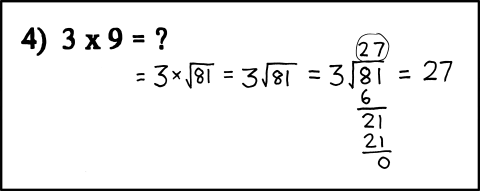759: 3x9

 3x9Title text: Handy exam trick: when you know the answer but not the correct derivation, derive blindly forward from the givens and backward from the answer, and join the chains once the equations start looking similar. Sometimes the graders don't notice the seam.

Explanation

In college courses with a very large number of students (picture the huge, tired, amphitheater-style lecture halls shown in any movie or TV show about college), teaching assistants are often employed to help the professors grade student work. In math and science courses, students are expected to solve the problems and show their work as supporting evidence. Due to the high volume of work to grade, whether it's being done by the professor or a TA, the grader will get lazy and look for correct answers and the existence of work without checking that the work is accurate.

The math shown in this comic switches from √ being square root notation to it being division notation midway. That is an illegal operation. But the correct answer is reached anyway, because 27 is the correct answer to 3 × 9, 3√81, and 81 ÷ 3.

More generally, this pattern holds true for any number and its square; namely, 𝑥𝑦 = 𝑦² ÷ 𝑥 whenever 𝑦 = x²   (... namely, x*y = y^2/x whenever y=x^2) .

The title text describes another technique usable when you remember the answer but not the calculations. It requires modifying the equation and the answer at the same time, hoping that at one point they'll look similar. Some students picture every step in the calculations, others skip some, as they seem obvious to them. Merging the equations once they look similar may trick the examiner into thinking that the step between them is obvious to the student, even if he IS checking the calculations. The side effect (not mentioned) is that while doing this, you may actually realize what the calculations should be.

Transcript

[A problem is given on an arithmetic test: "4) 3x9=?". In handwriting, the student's work follows. The student has accurately reformatted the question as 3 times the square root of 81, which visually resembles the long division problem of 3 divided into 81, and then solved the latter to get 27 — the correct answer to both.]add a comment! ⋅add a topic (use sparingly)! ⋅refresh comments!

Discussion

In the middle of a Physics I exam, I forgot one of the equations of motion. Using my basic working knowledge of Calculus and the relationship between acceleration, velocity, and position, I managed to derive an equation which I used to solve the problem. When I got my exam back, I was given only partial credit because I got the right answer using the wrong formula.Smperron (talk)

Ah, [insert your nation here]'s educational system at work. ImVeryAngryItsNotButter (talk) (please sign your comments with ~~~~)
I did something like this last year when we had to calculate how long it would take for something to fall ... meters on the moon, making a graph with y=v and x=t and making an integral by rewriting v to ...t^2, and then solving for the ... meters. My teacher gave me one point less for not using the correct formula but 2 bonus points for taking thinking about this solution. He is great. 13:37 UTC(I think) 8 octobre 2016
Or perhaps re-estimate the value of making the cubed root of 81 look like 27 when the marker knows it is really 4.32674871092 and a bit. I used Google News BEFORE it was clickbait (talk)
Uh, no, xth root is written as superscript....? Or am I missing the joke. 173.245.54.19 19:54, 31 October 2015 (UTC)

I had an old math teacher once who didn't spend too much effort in grading trickier problems, so I got away with something similar in deriving Lagrange's Trig Identity in a complex class. Maybe 8 steps from the LHS and 2 steps from the RHS were right, and the equals sign that joined them was a leap of faith. --Quicksilver (talk) 01:55, 20 August 2013 (UTC)

This is particularly useful when Wolfram|Alpha tells you the answer, but you don't know how to get the answer, and you can't afford Pro. MonacleforSauron (talk) 23:58, 1 August 2016 (UTC)

Some browsers seem not to support formulas without add-ons etc. I added the formula also in simple characters. 162.158.91.219 (talk) (please sign your comments with ~~~~)